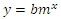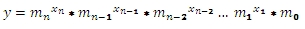# SQL Server GROWTH function

GROWTHMX__q

Updated: 15 November 2011

Use GROWTHMX_q to calculate values along an exponential trend. GROWTHMX_q is designed specifically for multiple x-values. If there is only one x-value for each y-value, consider using the aggregate function GROWTH.
GROWTHMX_q calculates the Ordinary Least Squares (OLS) solution for the given x- and y-values. The OLS solution calculates a regression analysis where an exponential curve is fitted. The known y-values are treated as dependent variables and the known x-values are treated as independent variables. For more information on the calculation of these values, see Ordinary Least Squares.
GROWTHMX_q computes a y-value with new x-values supplied as input to the function, using the coefficients calculated in the OLS solution.
In the case where we have one column of x-values and a column of y-values, the OLS solution is:For purposes of multi-linear regression, where there are multiple columns of x-values (and still a single column of y-values), the formula for the solution is described by the following equation:Where n is the number of x-columns and m0 is b.
The function expects the input to be in row-column, or spreadsheet, format, rather than in third-normal form. Thus, the input into the function requires the specification of the column names for the x-values and the y-values, and the specification of a 1-based index identifying the column reference for the y-values, and the specification of new x–values in the same column order as the x-values. Unlike a spreadsheet, however, there is no requirement that the columns containing the x-values be contiguous. The column specifications and the table or view that contains the data are passed into the function as strings.
Syntax
SELECT [wctStatistics].[wct].[GROWTHMX_q] (
<@Matrix_RangeQuery, nvarchar(4000),>
,<@y_ColumnNumber, int,>
,<@new_x, nvarchar(4000),>
,<@Lconst, bit,>)
Arguments
@MatrixRangeQuery
The SELECT statement, as a string, which, when executed, creates the resultant table of x- and y-values which will be used in the calculation. @MatrixRangeQuery values must evaluate to a type of float or of a type that implicitly converts to float.
@y_ColumnNumber
the index into the array identifying the column containing the y-values. The index value must be between 1 and n, where n is the number of columns specified in @ColumnNames. @y_ColumnNumber must be of the type int or of a type that implicitly converts to int.
@new_x
The new x-values for which you want TRENDMX to return a corresponding y-value. @new_x values must evaluate to a type of float or of a type that implicitly converts to float.
@LConst
A logical value specifying whether to force the y-intercept value (m0) equal to one. @LConst must be of the type bit or of a type that implicitly converts to bit.
Return Types
float
Remarks
·         If @Lconst is NULL than @Lconst is set to 'True'.
·         If @Lconst is true than the number of rows must be greater than the number of columns.
·         If @Lconst is false than the number of rows must be greater than or equal to the number of columns.
·         For simpler queries, you can try the GROWTHMX function.
Examples
We create a table and put some data in it.
CREATE TABLE #xy (
y     float,
x1    float,
x2    float,
x3    float
)

INSERT INTO #xy VALUES (64.744,-2,-8,0)
INSERT INTO #xy VALUES (115.589,8,3,-2)
INSERT INTO #xy VALUES (35.349,1,4,-7)
INSERT INTO #xy VALUES (77.215,-3,-1,1)
INSERT INTO #xy VALUES (28.725,-1,-3,10)
INSERT INTO #xy VALUES (54.679,5,-4,-7)
INSERT INTO #xy VALUES (27.382,-7,5,8)
INSERT INTO #xy VALUES (49.833,-8,-5,2)

This is what the data look like.
y                     x1                     x2                     x3
---------------------- ---------------------- ---------------------- ----------------------
64.744                     -2                     -8                      0
115.589                      8                      3                     -2
35.349                      1                      4                     -7
77.215                     -3                     -1                      1
28.725                     -1                     -3                     10
54.679                      5                     -4                     -7
27.382                     -7                      5                      8
49.833                     -8                     -5                      2
We have 3 columns of x-data and one column of y-data, which is in the first column of the table #xy. To invoke the table-valued function GROWTHMX_q, we enter the following SQL.
SELECT wct.GROWTHMX_q('SELECT y,x1,x2,x3 from #xy',1,'-7,9,-1','True') as GROWTH
The function is dynamically creating a SQL statement to execute @MatrixRangeQuery. This means that we can actually simplify this parameter, since we are selecting all the columns in this particular table, by entering the following:
SELECT wct.GROWTHMX_q('SELECT * from #xy',1,'-7,9,-1','True') as GROWTH
The second parameter, the y-column value, specifies that the 1st column in the resultant table, which becomes the input into the calculation, contains the y-values. The third parameter is the new x-vlaues, which is entered as a comma-separated string. The last parameter specified that we want to calculate the y-intercept.
The following result is produced.
GROWTH
----------------------
31.5136692835841
By looking at the LOGEST function, we can see how GROWTHMX_q came up with this value. If we run the following SQL, we can mimic the GROWTH calculation:
SELECT *
,CASE
WHEN idx = 0 THEN 1
WHEN idx = 1 THEN -7
WHEN idx = 2 THEN 9
WHEN idx = 3 THEN -1
END as new_x
FROM wct.LOGEST('#xy','*','',NULL,1,'True')
WHERE stat_name = 'm'
This returns the following result.
stat_name          idx               stat_val       new_x
---------- ----------- ---------------------- -----------
m                    0       51.5115125618439           1
m                    1       1.03997667832838          -7
m                    2      0.974365465751811           9
m                    3      0.983415556512974          -1
With a slight modification to the SQL and using the XLeratorDB PRODUCT function, we can now reproduce the same result as GROWTHMX_Q.
SELECT wctMath.wct.PRODUCT(POWER(stat_val, new_x)) as GROWTH
FROM (
SELECT *
,CASE
WHEN idx = 0 THEN 1
WHEN idx = 1 THEN -7
WHEN idx = 2 THEN 9
WHEN idx = 3 THEN -1
END as new_x
FROM wct.LOGEST('#xy','*','',NULL,1,'True')
WHERE stat_name = 'm'
) n
This produces the following result.
GROWTH
----------------------
31.5136692835842
Which is the same result returned by GROWTHMX_q
In this example, we will add a new table, containing multiple new x-values, demonstrating how to calculate multiple y-values from multiple new x-values in a single SELECT.
CREATE TABLE #new_x (
r     float,
x0    float,
x1    float,
x2    float
)

INSERT INTO #new_x VALUES (1,1,1,1)
INSERT INTO #new_x VALUES (2,2,2,-2)
INSERT INTO #new_x VALUES (3,3,-3,-3)
INSERT INTO #new_x VALUES (4,-4,-4,-4)
INSERT INTO #new_x VALUES (5,-5,5,-5)
We can then run the following statement and return a new y-value for each set of new x-values.
SELECT #new_x.r
,wct.GROWTHMX_q('SELECT * FROM #xy',1,cast(x0 as varchar)+','+cast(x1 as Varchar)+','+cast(x2 as varchar),'TRUE') as y
FROM #new_x
This produces the following result.
r                      y
---------------------- ----------------------
1       51.3318432932475
2       54.6916619602516
3       65.8564372652546
4       52.2365003841889
5       40.4306148196581
We also could have entered the following statement, using a SELECT statement to get the new x-values.
SELECT #new_x.r
,wct.GROWTHMX_q('SELECT * FROM #xy',1,'SELECT x0, x1,x2 from #new_x where r = ' + cast(#new_x.r as varchar),'TRUE') as y
FROM #new_x
Finally, if we wanted to calculate the coefficients without a y-intercept, and supplying some different new x-values, we would modify function call to make the last parameter FALSE, as seen in the following example.
SELECT wct.GROWTHMX_q('SELECT * from #xy',1,'10,10,7','False') as GROWTH
This produces the following result.
GROWTH
----------------------

0.0780313868197243

### SupportCopyright 2008-2023 Westclintech LLC         Privacy Policy        Terms of Service